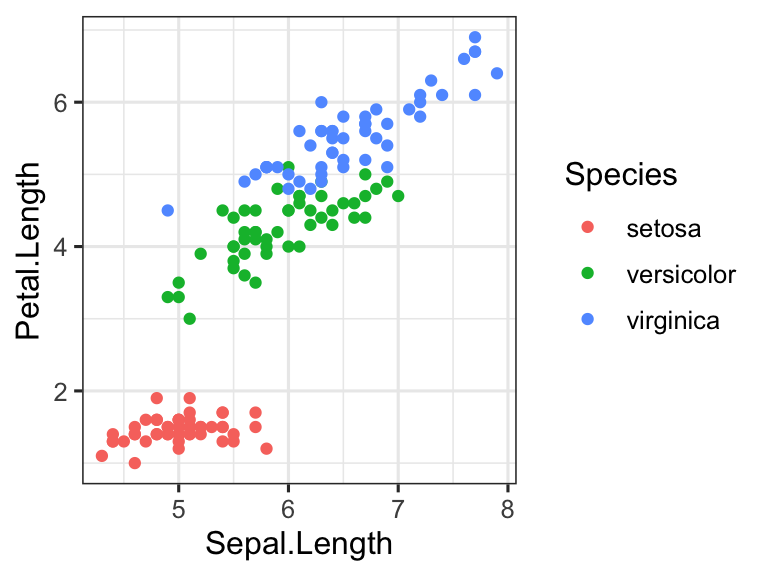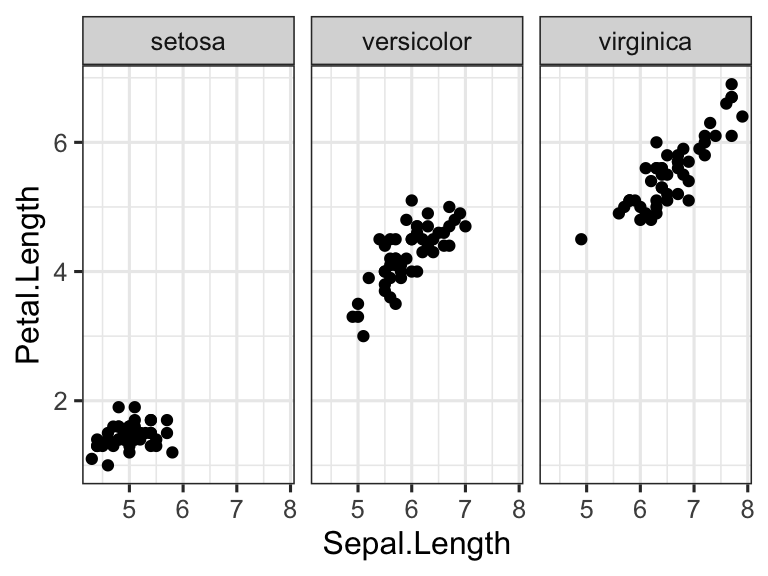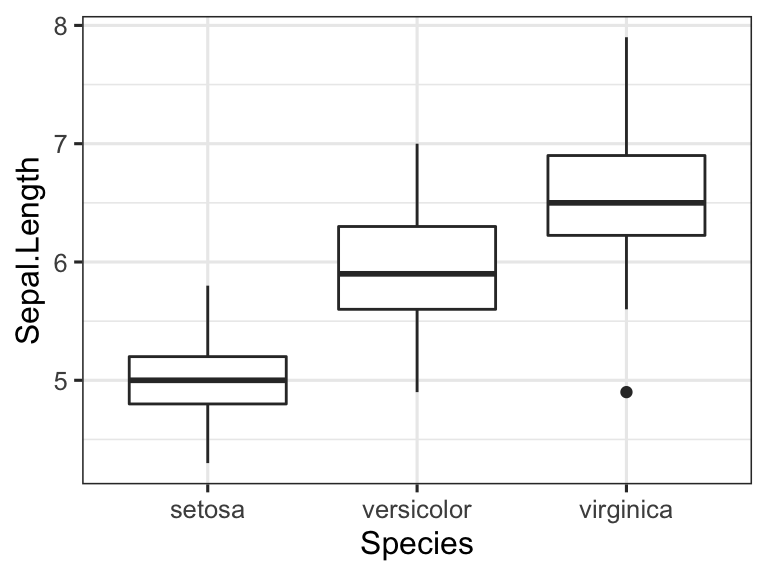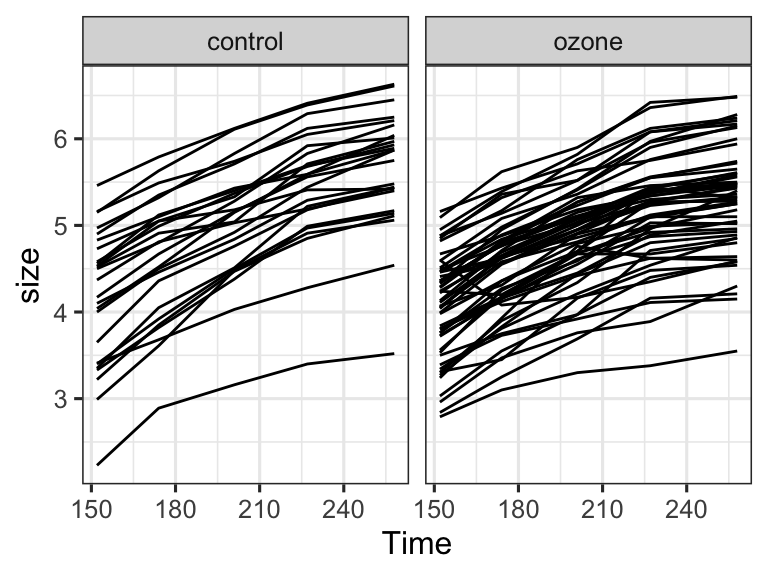Jan 29, 2019

## 1. Plotting the iris data set

We will work with the `iris` data set available in R. This data set gives the measurements in centimeters of the variables sepal length and width and petal length and width, respectively, for 50 flowers from each of 3 species of iris. The species are Iris setosa, versicolor, and virginica:

``head(iris)``
``````##   Sepal.Length Sepal.Width Petal.Length Petal.Width Species
## 1          5.1         3.5          1.4         0.2  setosa
## 2          4.9         3.0          1.4         0.2  setosa
## 3          4.7         3.2          1.3         0.2  setosa
## 4          4.6         3.1          1.5         0.2  setosa
## 5          5.0         3.6          1.4         0.2  setosa
## 6          5.4         3.9          1.7         0.4  setosa``````

In this worksheet, we will work with the library ggplot2, so we need to load it. We also set a theme that doesn’t use a gray background grid:

``````library(ggplot2) # load ggplot2 library
theme_set(theme_bw(base_size=12)) # set the default plot theme for the ggplot2 library``````

Using ggplot, make a scatter plot of petal length vs. sepal length for the three species. Then do the same plot but facet by species instead of coloring.

``ggplot(iris, aes(x=Sepal.Length, y=Petal.Length, color=Species)) + geom_point()````ggplot(iris, aes(x=Sepal.Length, y=Petal.Length)) + geom_point() + facet_wrap(~Species)``Now make side-by-side boxplots of sepal lengths for the three species of iris. The geom you need to use is `geom_boxplot()`. See if you can guess the correct aesthetic mapping.

``ggplot(iris, aes(y=Sepal.Length, x=Species)) + geom_boxplot() ``## 2. Plotting tree-growth data

The data set `sitka` contains repeated measurements of tree size for 79 Sitka spruce trees, which were grown either in ozone-enriched chambers or under control conditions. It contains four columns: `size` measures the size of the tree (height times diameter squared, on a log scale). `Time` measures the time, in days since Jan. 1, 1988. `tree` indicates the tree we are working with, consecutively numbered from 1 to 79. `treat` indcates the treatment trees were subjected to, either `ozone` for an ozone-enriched chamber or `control`.

``````# download the sitka data set:
sitka <- read.csv("http://wilkelab.org/classes/SDS348/data_sets/sitka.csv")
head(sitka)``````
``````##   size Time tree treat
## 1 4.51  152    1 ozone
## 2 4.98  174    1 ozone
## 3 5.41  201    1 ozone
## 4 5.90  227    1 ozone
## 5 6.15  258    1 ozone
## 6 4.24  152    2 ozone``````

Make line plots of tree size vs. time, for each tree, faceted by treatment. First, use the same color for all lines. Hint: you will need to use the `group` aesthetic to tell ggplot that you want to have a separate line for each tree.

``ggplot(sitka, aes(x=Time, y=size, group=tree)) + geom_line() + facet_wrap(~treat)``Now, make a variant of this plot where each tree has a separate color.

``ggplot(sitka, aes(x=Time, y=size, color=tree, group=tree)) + geom_line() + facet_wrap(~treat)``